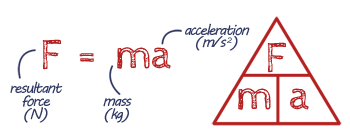# Force and Motion

## Key Words

Newton's First Law

If the resultant force on a stationary object is zero, the object will remain stationary.

If the resultant force on a moving object is zero, it'll just carry on moving at the same velocity.

Resultant Force - the overall force on a point or object.

Mass - the amount of 'stuff' in a object.

Weight - the force acting on an object due to gravity.

Gravitational Force - the force of attaction between masses.

Newton's Second LawInertia - when an object stays in the same state of motion.

Newton's Third Law

When two objects interact, the forces they exert on each other are equal and opposite.

Equilibrium - when the forces on an object are balanced.

Friction - the force resisting  movement between objects.

Reaction time - the time it takes for a person think see something then do something.

Momentum - how much 'oomph' an object has.

Also see Forces, Motion, Force & Pressure.

## Revision NotesPrint out revision notes

Need more paper?
Print more

## EQUATION Resultant Force (Newton's Second Law of Motion)## EQUATION Momentum## PRACTICAL Investigating force & acceleration

Watch the video and have a go at writing out the steps of the practical.

Note paper for practical